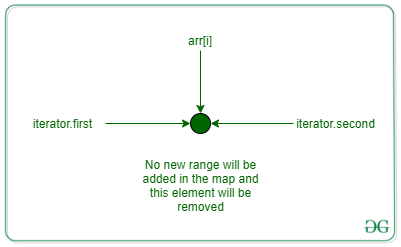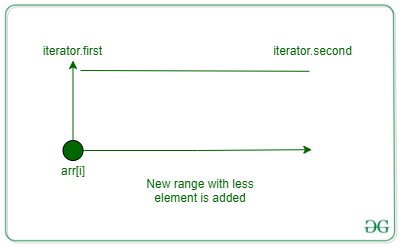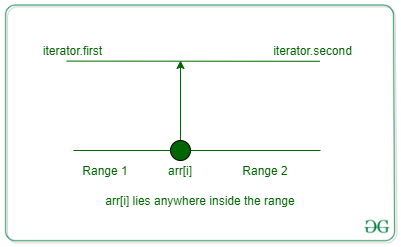# Final Exam | Google Kickstart 2021 Round D

• Difficulty Level : Hard
• Last Updated : 21 Sep, 2021

It’s time for the final exam in algorithms and data structures!

Edsger prepared N sets of problems. Each set consists of problems in an increasing difficulty sequence; the i-th set can be described by two integers Ai and Bi (Ai≤Bi), which denotes that this set contains problems with difficulties Ai, Ai+1…, Bi. Among all problems from all sets, it is guaranteed that no two problems have the same difficulty.

This semester Edsger has to test M students. He wants to test each student with exactly one problem from one of his sets. No two students can get the exact same problem, so when Edsger tests a student with some problem, he cannot use this problem anymore. Through countless lectures, exercises, and projects, Edsger has gauged student number j to have skill level Sj and wants to give that student a problem with difficulty Sj. Unfortunately, this is not always possible, as Edsger may have not prepared a problem of this difficulty, or he may have already asked this problem to some other student earlier. Therefore, Edsger will choose for the j-th student a problem of difficulty Pj, in a way that |Pj−Sj| is minimal and a question of difficulty Pj was not already given to any of the students before the j-th student. In case of ties, Edsger will always choose the easier problem. Note that the problem chosen for the j-th student may affect problems chosen for all the students tested later, so you have to process students in the same order as they appear in the input.

As keeping track of all the problems can be fairly complicated, can you help Edsger and determine which problems he should give to all of his students?

OR

Given an array problemRange of pairs having starting and ending values as a range of difficulty levels, and an array arr of size M indicating the difficulty level every student can attempt. The task is to assign a unique integer X from problemRange to every integer in array arr such that | arr[i] – X | is minimized. In case of a tie between two values closest to arr[i], a lesser difficulty value must be chosen. X values must be assigned to students in their order since the same value of X cannot be assigned to more than one student. Print the X value assigned to every student.

Example:

Input: N = 5, M = 4, arr = [14, 24, 24, 4], problemRange = [[1, 2], [6, 7], [9, 12], [24, 24], [41, 50]]
Output: 12 24 11 2
Explanation: values which can be assigned to the students are {1, 2}, {6, 7}, {9, 10, 11, 12}, {24}, {41, 42, 43, 44, 45, 46, 47, 48, 49, 50} 12 is assigned to first student who can attempt questions of difficulty level 14 as it is the closest to 14. 24 is closest to 24. Next student can also attempt question of 24 difficulty but 24 from the range is already chosen and the next closest is 11. 2 and 6 is closest to last student of difficulty 4, since 2 and 6 both are similarly close to 4, easier questions of difficulty level 2 is assigned.

Input: N = 1, M = 1, arr = , problemRange = [[42, 42]]
Output: 42

Approach: Given problem can be solved by following the steps below:

• Use a map to store the start of ranges as keys and end of ranges as values
• Iterate the array and for every element in it find its lower_bound in the map
• Two cases are possible: Lower_bound will return the iterator pointing to the key which will be equal to arr[i] or the key which is just greater than arr[i]
• let’s say the iterator provided by lower_bound be it and pre be an iterator just before it (pre will be equal to it when it=mp.begin())
• Either pre.first<=arr[i]<=pre.second or it.first<=arr[i]<=it.second will be true. arr[i] will lie in either the forward section or in the backward section of this range
• every time value is assigned to the arr[i] previous range is deleted from the map and a new range is added as shown in the image
• Either two new ranges are added or one new range is added as cases shown in the images below:Only one element is present in the range so it is removed and no new range is addedarr[i] is equal to either one of the rangeThe previous range is removed and two new ranges are added in the map

Below is the implementation of the above approach:

## C++

 `// C++ implementation for the above approach` `#include ` `using` `namespace` `std;` `void` `solve(``long` `long` `int` `N, ``long` `long` `int` `M,``           ``vector >``               ``problemRange,``           ``vector<``long` `long` `int``> arr)``{` `    ``// Store the problem range in a map``    ``map<``long` `long` `int``, ``long` `long` `int``> mp;` `    ``for` `(``long` `long` `int` `i = 0; i < N; i++) {``        ``long` `long` `int` `a, b;``        ``a = problemRange[i].first;``        ``b = problemRange[i].second;``        ``mp[a] = b;``    ``}` `    ``vector<``long` `long` `int``> ans(M);` `    ``for` `(``long` `long` `int` `i = 0; i < M; i++) {``        ``auto` `it = mp.lower_bound(arr[i]);``        ``auto` `pre = it;``        ``if` `(it != mp.begin())``            ``pre--;` `        ``// If answer lies in a valid range``        ``if` `(pre->first <= arr[i]``            ``&& arr[i] <= pre->second) {``            ``ans[i] = arr[i];``            ``long` `long` `int` `st = pre->first,``                          ``end = pre->second;``            ``mp.erase(pre);``            ``long` `long` `int` `left = arr[i] - 1,``                          ``right = arr[i] + 1;``            ``if` `(st <= left) {``                ``mp[st] = left;``            ``}``            ``if` `(end >= right) {``                ``mp[right] = end;``            ``}``        ``}` `        ``// If answer is not in a valid range``        ``else` `{``            ``long` `long` `int` `op1 = pre->second,``                          ``op2 = it->first;``            ``if` `(``abs``(arr[i] - op1) <= ``abs``(arr[i] - op2)) {``                ``ans[i] = op1;``                ``long` `long` `int` `st = pre->first,``                              ``end = op1 - 1;``                ``mp.erase(pre);``                ``if` `(st <= end)``                    ``mp[st] = end;``            ``}``            ``else` `{``                ``ans[i] = op2;``                ``long` `long` `int` `st = it->first + 1,``                              ``end = it->second;``                ``mp.erase(it);``                ``if` `(st <= end)``                    ``mp[st] = end;``            ``}``        ``}``    ``}``    ``for` `(``auto` `it : ans)``        ``cout << it << ``" "``;``    ``cout << endl;``}` `// Driver code``int` `main()``{``    ``long` `long` `int` `N, M;``    ``N = 5;``    ``M = 4;` `    ``// Student difficulty level``    ``vector<``long` `long` `int``> arr = { 14, 24, 24, 4 };` `    ``vector >``        ``problemRange = { { 1, 2 },``                         ``{ 6, 7 },``                         ``{ 9, 12 },``                         ``{ 24, 24 },``                         ``{ 41, 50 } };``    ``solve(N, M, problemRange, arr);``    ``return` `0;``}`
Output
`12 24 11 2 `

Time complexity: MLog(N)
Auxiliary Space: O(N)

My Personal Notes arrow_drop_up